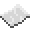# table.sort

Sort the array, using the function sorter to compare the elements, or `<` if it is not given, in place. If no sorter is specified, the array must consist entirely of comparable values (either numbers or strings, but not both).ExampleSort an array
Print a sorted version of the array, which is turned into a string using table.concat.
Code
```<nowiki>
t = { 5, 4, 2, 1, 3 }
table.sort(t)
print(table.concat(t, ', '))
</nowiki>
```
Output
```1, 2, 3, 4, 5
```ExampleFind the smallest element in an array of random numbers
Instead of using a for loop to find the smallest element in an array, it is possible to sort and then index it. The first index will be the smallest element.
Code
```<nowiki>
local elements = 10
local array = {}
for i = 1, elements do
array[i] = math.random(1, 1000)
end
table.sort(array)
print(array[i])
</nowiki>
```
Output The smallest number in `array`, which depends on how the array was filled.ExampleSort an array of tables by their length
This demonstrates using a custom sorter function to compare tables by their length. Note that generally `<` will not compare tables.
Code
```local array = { { 'foo', 'bar', 'baz' }, { 'red', 'green', 'blue' }, { 'apple', 'orange' } }
table.sort(array, function(a, b)
return #a < #b
end)
print(array)
```
Output
```'apple'
```

table.sort
Function
Syntax
table.sort(
• table : table
• sorter? : function
)

Returns nil
API table
Source Lua (source)